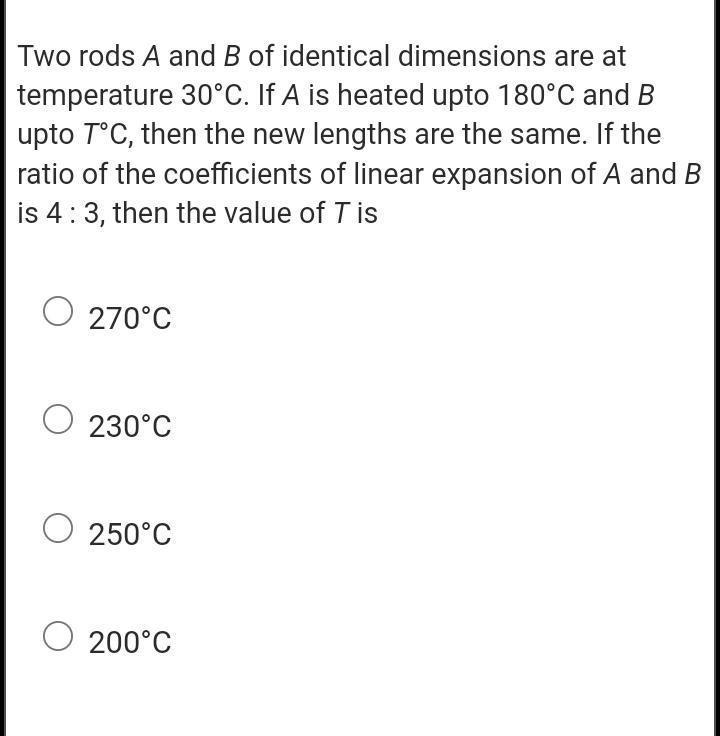Question:

# Two rods A and B of identical dimensions are at temperature 30°C. If A is heated upto 180°C and B upto T°C, then the new lengthsTwo rods A and B of identical dimensions are at temperature 30°C. If A is heated upto 180°C and B upto T°C, then the new lengths are the same. If the ratio of the coefficients of linear expansion of A and B is 4:3, then the value of T is O 270°C O 230°C 250°C 200°C